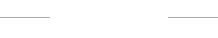• VIP会员• 注册
• 登录
• 公众号深圳住朋网微信公众号

• 小程序住朋网

随时随地了解房市住朋帮经纪人

客户裂变，房源海报，房源发布，在线聊天，分销报备，精准拓客。住购宝

全民经纪人，注册方便，放心赚佣

• 住朋网APP随时随地了解房市

• 住朋云

• 全部
• 龙岗
• 龙华
• 宝安
• 南山
• 福田
• 罗湖
• 坪山
• 盐田
• 大鹏新区
• 光明新区
• 惠州
• 东莞

• 不限
• 1号线
• 2号线
• 3号线
• 4号线
• 5号线
• 7号线
• 9号线
• 11号线

• 不限
• 2万以下
• 2-3万
• 3-4万
• 4-5万
• 5-6万
• 6-7万
• 7-8万
• 8-9万
• 9-10万
• 10万以上
• 确定

• 不限
• 住宅
• 别墅
• 商铺
• 写字楼
• 酒店

• 不限
• 待售
• 在售
• 售罄

7-8万 删除全部条件

• 龙岗
•  ├坪地
•  ├坂田
•  ├双龙
•  ├横岗
•  ├布吉
•  ├平湖
•  ├大运新城
•  ├丹竹头
•  ├龙岗中心城
•  ├宝荷
•  ├龙东
• 龙华
•  ├民治
•  ├观澜
•  ├龙华中心
•  ├上塘
•  ├红山
•  ├梅林关
•  ├龙华新区
• 宝安
•  ├西乡
•  ├桃源居
•  ├新安
•  ├宝安中心
•  ├石岩
•  ├曦城
•  ├福永
•  ├沙井
•  ├翻身
•  ├碧海
•  ├尖岗山
•  ├松岗
• 南山
•  ├蛇口
•  ├科技园
•  ├前海
•  ├红树湾
•  ├大学城
•  ├西丽
•  ├深圳湾
•  ├华侨城
•  ├南山中心
•  ├南头
•  ├后海
•  ├白石洲
• 福田
•  ├香蜜湖
•  ├皇岗
•  ├梅林
•  ├华强南
•  ├华强北
•  ├上步
•  ├福田中心
•  ├景田
•  ├石厦
•  ├竹子林
•  ├银湖
•  ├八卦岭
•  ├福田保税区
•  ├莲花
•  ├百花
•  ├上下沙
•  ├车公庙
•  ├园岭
•  ├黄木岗
•  ├新洲
•  ├赤尾
•  ├沙尾
•  ├香梅北
• 罗湖
•  ├地王
•  ├黄贝岭
•  ├万象城
•  ├莲塘
•  ├银湖
•  ├洪湖
•  ├罗湖口岸
•  ├布心
•  ├翠竹
•  ├东门
•  ├笋岗
•  ├春风路
•  ├百仕达
•  ├清水河
•  ├ 螺岭
•  ├新秀
• 坪山
• 盐田
•  ├梅沙
•  ├沙头角
•  ├盐田港
• 大鹏新区
• 光明新区
•  ├公明
•  ├光明
• 惠州
• 东莞

下载住朋网APP• 0

楼盘对比最多对比5个•住朋移动微官网